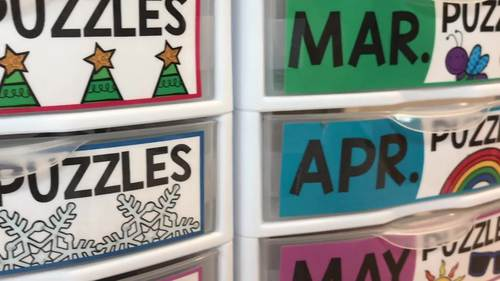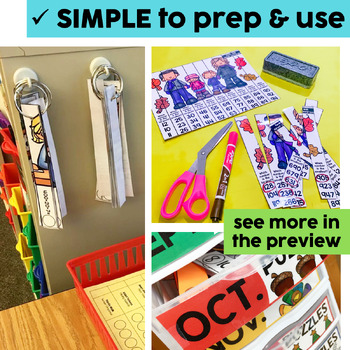# Math Puzzles | Math Centers, Games & Activities | Valentine's Day ActivitiesSubject
Resource Type
File Type

Zip

(194 MB|800+)
Product Rating
Standards
• Product Description
• StandardsNEW
Math Puzzles are a great option for your early finishers, math centers, enrichment opportunities, morning work, or even intervention groups. Each puzzle focuses on a math skill. There are 10 pieces to each puzzle. Each puzzle piece has a math problem for the student to solve. Then the student assembles the puzzle by putting their pieces in order from least to greatest.

These puzzles also come with a student checklist. Each puzzle has a title in the upper right hand corner. This is an easy way for the student to identify the puzzle on his/her checklist.

***UPDATE: There is now a monthly math puzzle student recording sheet included in this bundle! Students write the name of the puzzle and the answers they got on the recording sheet. We've also added an editable recording sheet as well!
Great for accountability and quick check assessment of skills!***

Each puzzle comes with four options for printing:
Option #1: full color puzzle (laminate, cut, write, & wipe)
Option #2: black and white puzzle (print on bright paper, laminate, cut, write, & wipe)
Option #3: student template (pieces mixed up for student to solve, cut, and assemble into a notebook or on a piece of construction paper)
Option #4: EDITABLE puzzle pieces for you to customize to meet the needs of your classroom

**************************************************************************

September Math Puzzles
The math skills covered in these puzzles are: addition to 20, subtraction within 20, counting coins, number rounding to 10, number rounding to 100, addition without regrouping, addition with regrouping, domino addition, multiplication, 10 more/10 less, 1 more/1 less, number bonds, number words 0-9, number words 10-19
Take a closer look at the September puzzles HERE!

October Math Puzzles
The math skills covered in these puzzles are: tens & ones, addition, base ten blocks, expanded form, money(penny, nickel, dime, and quarter), subtraction, missing addends, number patterns, number sequencing, and least to greatest.
Take a closer look at the October puzzles HERE!

November Math Puzzles
The math skills covered in these puzzles are: expanded form (2-digit, 3-digit, and 4-digit), basic addition facts, basic multiplication facts, odd and even (1-digit and 2-digit), value of digits (2-digit and 3-digit), missing addends (1-digit and 2-digit), number comparisons (2-digit and 3-digit), 2-digit addition (with and without regrouping), adding three numbers (1-digit and 2-digit), counting money (penny, nickel, dime, and quarter), base ten blocks (ones, tens, and hundreds), make a ten/missing addends using a ten frame, least to greatest
Take a closer look at the November puzzles HERE!

December Math Puzzles
The math skills covered in these puzzles are: addition, subtraction, multiplication, time to the hour, place value (value of the underlined digit), plus and minus 1 & 10 boxes, counting coins (penny, nickel, dime, & quarter), missing addends with doubles, using doubles to subtract, fact families, skip counting (by 2's, 3's, 5's, & 10's), expanded form (2-digit and 3-digit), number bonds, 2-digit addition, 2-digit subtraction, and least to greatest
Take a closer look at the December puzzles HERE!

January Math Puzzles
The math skills covered in these puzzles are: 2-Digit Subtraction (with and without regrouping), Part Part Whole, Time to the Half Hour, Fractions, Multiplication, 2-Digit Addition (with and without regrouping), Counting Coins, Doubles Facts-Addition, Subtraction, Skip Counting by 5's, Number Bonds, 1 more/1 less, 10 more/10 less, and least to greatest
Take a closer look at the January puzzles HERE!

February Math Puzzles
The math skills covered in these puzzles are: Addition, Subtraction, Multiplication, 1 More/1 Less and 10 More/10 Less Number Boxes, Counting Coins (penny, nickel, dime, quarter, and half dollar), Place Value (value of underlined digit- 2- and 3-digit numbers), Fact Families, Number Bonds, 2-Digit Addition (with and without regrouping), 2-Digit Subtraction (with and without regrouping), Part Part Whole (missing part), Telling Time to the Nearest 5 Minutes, Rounding (to nearest 10 and 100), Least to Greatest
Take a closer look at the February puzzles HERE!

March Math Puzzles
The math skills covered in these puzzles are: Rounding Numbers (nearest 10 & 100), 2-Digit Addition (with & without regrouping), Addition, Subtraction, Multiplication, 1 More/1 Less, 10 More/10 Less, Place Value, Adding Three Numbers, Counting Coins (penny, nickel, dime, quarter, & half dollar), Place Value Number Boxes, Missing Addends
Take a closer look at the March puzzles HERE!

April Math Puzzles
The math skills covered in these puzzles are: Addition, Subtraction, Multiplication, 2-Digit Addition (with regrouping), 2-Digit Addition (without regrouping), Number Bonds, Telling Time (nearest 5 minutes), Adding Three Numbers (1- and 2-digit), Expanded form (2- and 3-digit), Fact Families, Missing Addends, Place Value Boxes, Counting Coins, 2-Digit Subtraction (with regrouping), 2-Digit Subtraction (without regrouping), Part Part Whole and Least to Greatest
Take a closer look at the April puzzles HERE!

May Math Puzzles
The math skills covered in these puzzles are: 3-Digit Addition, 3-Digit Subtraction, Adding 3 Numbers (2-digit numbers), Digit Values (2-digit and 3-digit numbers), Counting Coins (penny, nickel, dime, quarter, & half dollar), Part Part Whole, Multiplication, Fact Families, Telling Time (telling time to the nearest 5 minutes), Fractions (halves, thirds, fourths, fifths, sixths, sevenths, eighths, ninths, and tenths), Base Ten Blocks (ones, tens, hundreds), Even & Odd (2-digit and 3-digit numbers), Place Value (2-digit and 3-digit numbers), and Least to Greatest
Take a closer look at the May puzzles HERE!

▼▼▼▼▼▼▼▼▼▼▼▼▼▼▼▼▼▼▼▼▼▼▼▼▼▼▼▼▼▼▼▼▼▼▼▼▼▼▼▼▼▼▼▼

If you would like to get updates on NEW and CURRENT resources...
FOLLOW me on Teachers Pay Teachers!
FOLLOW the Lucky Little Learners Blog!

▼▼▼▼▼▼▼▼▼▼▼▼▼▼▼▼▼▼▼▼▼▼▼▼▼▼▼▼▼▼▼▼▼▼▼▼▼▼▼▼▼▼▼▼

Permission to copy for single classroom use only.
Electronic distribution limited to single classroom use only.
Not for public display.

Partition circles and rectangles into two, three, or four equal shares, describe the shares using the words halves, thirds, half of, a third of, etc., and describe the whole as two halves, three thirds, four fourths. Recognize that equal shares of identical wholes need not have the same shape.
Determine whether a group of objects (up to 20) has an odd or even number of members, e.g., by pairing objects or counting them by 2s; write an equation to express an even number as a sum of two equal addends.
Fluently add and subtract within 20 using mental strategies. By end of Grade 2, know from memory all sums of two one-digit numbers.
Mentally add 10 or 100 to a given number 100–900, and mentally subtract 10 or 100 from a given number 100–900.
Add and subtract within 1000, using concrete models or drawings and strategies based on place value, properties of operations, and/or the relationship between addition and subtraction; relate the strategy to a written method. Understand that in adding or subtracting three-digit numbers, one adds or subtracts hundreds and hundreds, tens and tens, ones and ones; and sometimes it is necessary to compose or decompose tens or hundreds.
Total Pages
800+
N/A
Teaching Duration
N/A
Report this Resource to TpT
Reported resources will be reviewed by our team. Report this resource to let us know if this resource violates TpT’s content guidelines.\$20.00
List Price:
\$36.00
You Save:
\$16.00